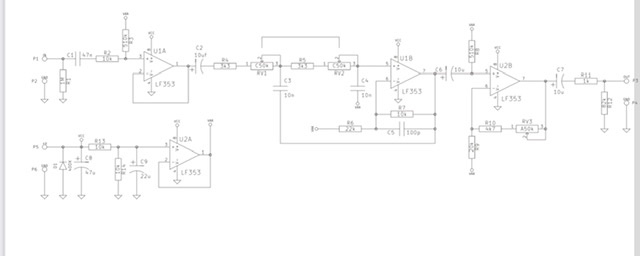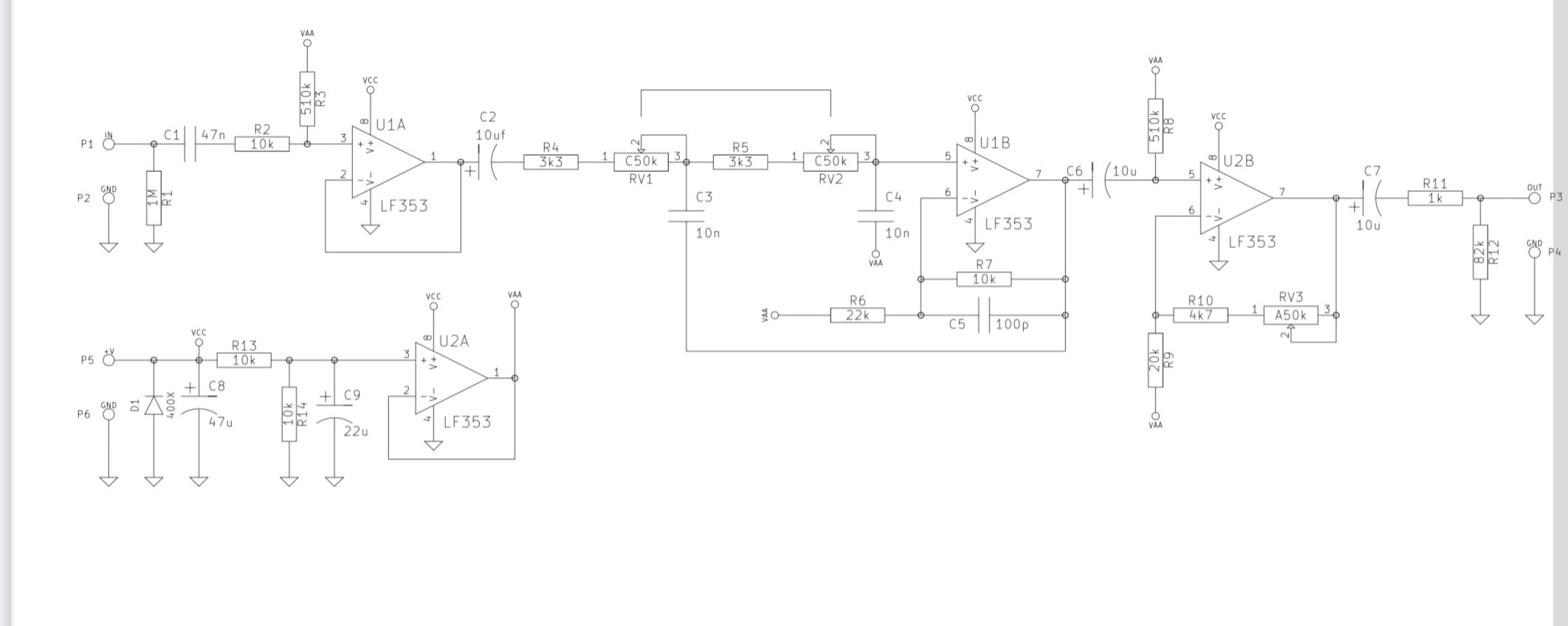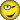# LPF questionClassicListThreaded21 messages12
Open this post in threaded view
|

## LPF question

 I was wondering if someone could tell me what type of LPF this is. Sallen 2nd order, inverting, non-inverting etc.. I’m looking to change the freq sweep and would like to run it through a lpf calculator but I’m unsure of what type it is.Open this post in threaded view
|

## Re: LPF question

 Do you have a bigger or easier to read image? I can't read this one very easily.
Open this post in threaded view
|

## Re: LPF question

 I will try this oneOpen this post in threaded view
|

## Re: LPF question

 Looks like Voltage-Controlled Voltage Source (VCVS) low-pass filter (2nd order, non-inverting), which is a variation on a Sallen-Key filter with gain. In case it's not obvious: - the top left op-amp is an input buffer - the bottom left op-amp is a buffer for the 1/2 rail reference voltage - the op-amp on the right is a makeup gain stage - the stuff between C2 and C6 is the filter Here's some links: https://en.wikipedia.org/wiki/Sallen%E2%80%93Key_topologyhttp://nicadd.niu.edu/~fortner/course/phys475/lect/p575_07b.pdfhttps://www.electronics-tutorials.ws/filter/second-order-filters.htmlhttps://electronics.stackexchange.com/questions/74877/gain-in-sallen-key-filterPlenty more with a google search if you're interested.
Open this post in threaded view
|

## Re: LPF question

 Thank you for the info. This is some good reading. I’ll take in as much as you want to share.
Open this post in threaded view
|

## Re: LPF question

 I’m not sure if I have this figured right. An I correct in thinking the C50k potentiometer figures in to the filter formula. Would the filter be comprised of 3k3 + 50k = R1 53.3k with C3 making the first filter @ 298.6 Hz. Seeing as though the info / links above don’t show a 1 to 1 diagram with the schematic I have , I’m having a little trouble putting it all together. I’m all about learning though,Open this post in threaded view
|

## Re: LPF question

 You've got it mostly right. The part of the circuit between c2 and c6 in your schematic is actually 1:1 with the relevant circuits in the links if you appropriately add the impedances in series and in parallel. I'll use uppercase letters for the formulae in the links, and lowercase letters for components in your schematic: For the corner frequency calculation, we have fc = 1/(2*pi*sqrt(R1*R2*C1*C2)) Comparing to your schematic, we have R1 = r4+rv1, R2 = r5+rv2, C1=c3, C2=c4. rv1 and rv2 both range from 0 to 50k, so fc ranges from 298.6 Hz when rv1=rv2=50k, to 4822.9 Hz when rv1=rv2=0. These are pretty low frequencies for a low-pass filter, so I'm guessing this circuit is for bass guitar or a preamp for upright bass or something similar. The gain is given by Av = 1+R3/R4. Without c5, the gain would be frequency-independent, but the impedance of a capacitor is given by Zc = 1/(2*pi*f*C), which is frequency-dependent. Another name for frequency dependent gain is EQ, so we really should account for c5 in the frequency calculations. This is really only a slight complication though because c5 is tiny. The impedance of a cap and resistor in parallel is |Z| = 1/sqrt(R^-2+(2*pi*f*C)^2), which is gives R3=10k at 20 Hz and 9.9k at 20 kHz. This gives a gain of 1.45 at all frequencies that humans can hear, which means we can ignore it for the purpose of calculating the corner frequency of the filter. (At f=160 kHz, the gain is about 1.3, and as the frequency approaches infinity, the gain slowly drops to 1. So c5 is probably just there to prevent oscillation at ultrasonic frequencies.) As a final note, I'll point out that the corner frequency calculations are symmetric with respect to R1, R2, C1, and C2. So most likely, rv1 and rv2 should a dual-gang pot rather than two separate pots, since the effect of both pots would be identical.
Open this post in threaded view
|

## Re: LPF question

 Thank you so much for taking the time to explain that. I pretty much understand, except for how to do the math formulas. I will research that more. RV1 & 2 are a dual gang C50k. I was close . I had the lower freq correct but was using the wrong C for C2 . With the aid of online calculators I may know just enough to get myself underway. The schematic is from a LPF for bass. Im not to keen on the frequencies they have it specd at thus the reason for this topic. Thanks again for your help, 52 and still learning, love it. Matt
Open this post in threaded view
|

## Re: LPF question

 My pleasure. If you need help customizing it to a specific frequency sweep, let me know.
Open this post in threaded view
|

## Re: LPF question

 Let’s see if I have figured this out correctly. If I leave R4 =3k3 + 0k ( min pot value) and change C3 to C3=2n2n,  cutoff freq = 21,992 If I leave R5= 3k3+50k (max pot value) and change C4 to 15n , cutoff freq = 199.06 Does this sound correct.
Open this post in threaded view
|

## Re: LPF question

 This post was updated on . If you keep both pots at 50k and change both c3 and c4 to 2n2, the corner frequency sweeps from 1.3 kHz to 21.9 kHz. If you keep both pots at 50k and change both c3 and c4 to 15n, the corner frequency sweeps from 199 Hz to 3.2 kHz.
Open this post in threaded view
|

## Re: LPF question

 So you need to keep both C3 and C4 at the same value?  I thought I could make them different to change the sweep. I have more reading to do I think. The 50k is the dual pot value . I was thinking that RV1, R4 and C3 made one part of the filter and RV2, R5 and C4 made the other part of the filter , I’m missing something. I will dig deeper. Ultimately I’m trying to get a 250hz to 22k sweep. I really need to learn this. Thanks again, Matt
Open this post in threaded view
|

## Re: LPF question

Open this post in threaded view
|

## Re: LPF question

 I’m in over my head here. I do not know how to calculate the formulas. I didn’t take that kinda of math, more of a geometry guy. I can somewhat understand them but not enough to calculate. I checked the specs on my bass cabinet and it has a range of 35Hz to 14Khz. My intention was to keep both pots at 50k, change c3 to 2n2 and change c4 to 15n but that doesn’t give me the results expected. I have been using an online passive RC LowPass filter calculator. So I’m only calculating 1 filter at a time. I assumed that the R/C and potentiometer worked independent of each other but now I see that’s not the case. I give up and humbly ask what are the values for the 250Hz 22Khz sweep. Maybe if I know the answer I can work backwards and figure it out for the future. I’m an old construction guy trying to learn something new. I did google how to calculate formulas but all that got me was a bunch of Excel spreadsheet stuff. Thanks again Matt
Open this post in threaded view
|

## Re: LPF question

 If you keep both pots at 50k, make r4=470, r5=680, c3=10n, and c4=15n, the corner frequency sweeps from 257 Hz to 23 kHz. There are other cap and resistor values that would work, but I chose these because they get you pretty close to the specified frequencies with standard components that should be easy to find. I don't think that you will be able to hear any difference after the corner frequency goes above 5k, though.
Open this post in threaded view
|

## Re: LPF question

 Thank you very much. This has been very educational. Hopefully I will be able to figure this out with some more time. You have given me a lot of food for thought and for that I deeply thank you. Knowledge is key. This project started as a LPF to use as a high cut for bass, I am a bass player and an avid DIYer. In your opinion what do you think would be the best sweep for this. 500Hz to 5k maybe? That would also be useful for guitar as well? I have seen another LPF diy kit project that I could just buy and build but there is no learning involved in that. This kit advertises the sweep being from 250Hz to 22K so that is where I came up with my sweep numbers. Thanks again, Matt
Open this post in threaded view
|

## Re: LPF question

 I haven’t given up yet, let’s see if I get this one right, lol. Leave pots at 50k Make R4=1k, R5=1K, C3=10n, C4=15n , corner frequency sweep = 254Hz to 13Khz.
Open this post in threaded view
|

## Re: LPF question

Open this post in threaded view
|

## Re: LPF question

 Great info. Thank you for taking the time to educate me. I have saved all the info to a folder for easy reference as needed. I have learned a lot and also learned I have a lot more to learn, lol. All the Best, Matt